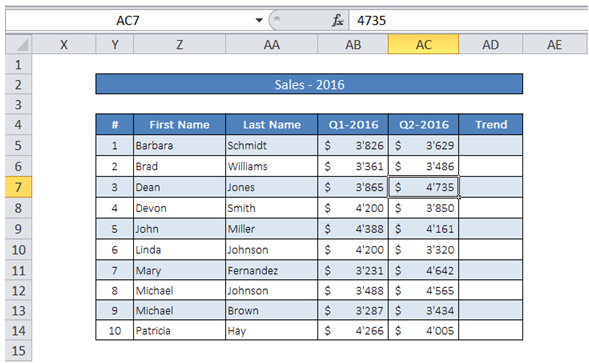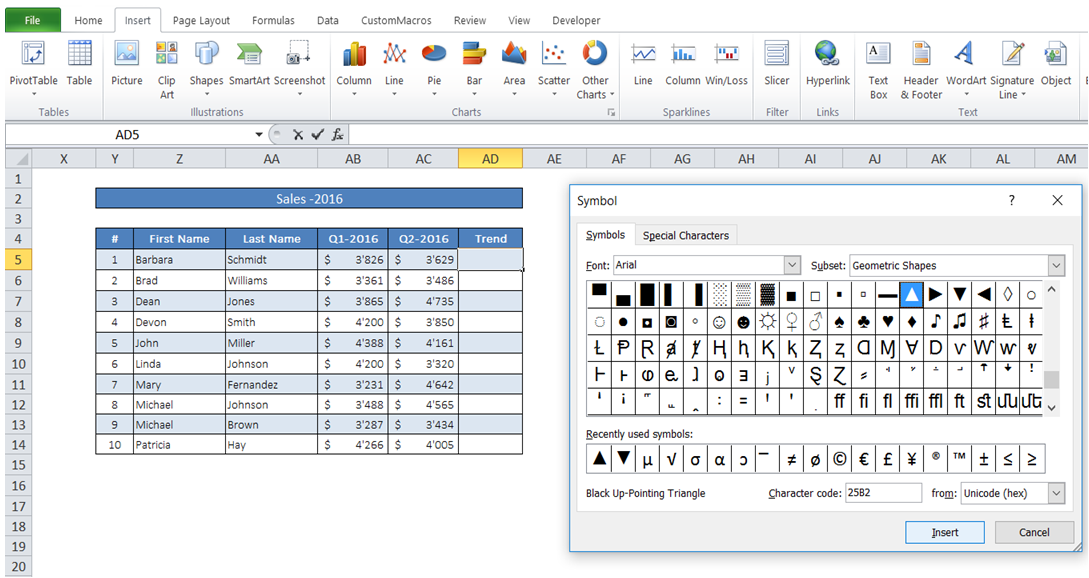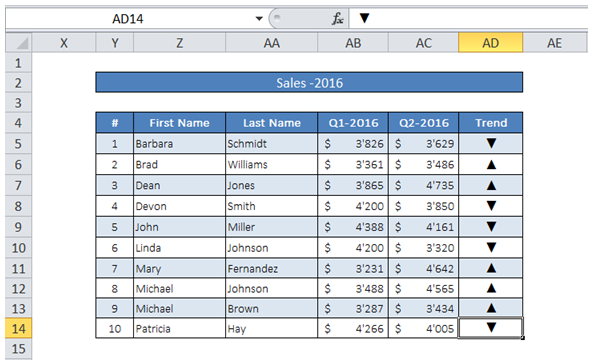## Vba insert triangle in cells in Excel

For example, I want to show whether Sales has increased or decreased compared to previous period using Triangle / Inverted Triangle - how can I add the same using VBA?To do it in Excel, here is the answer:

a) First the character code for Triangle and Inverted Triangle should be obtained. For that, click on "Symbol" under "Insert" as shown below. In the "Symbol" dialog box click on the Symbol of choice and note down the Character code.

In this case it is Hex 25B2 for Triangle and 25BC for Inverted Triangle.a) Click on any cell in the Table. In the Ribbon, "Table Tools" Design tab appears. Click on "Total Row" under "Table Style Options". "Total Row" appears at the end of the Table.

b) Use the Hex codes to fill the cell appropriately with Triangle or Inverted Triangle using ChrW(CharCode in Hex) function after applying the condition using VBA (see macro below).

1. Option Explicit
2. Sub InsertSymbols()
3. Dim i As Integer
4. '''
5. 'Loop through Table
6. For i = 5 To 14
7. If ActiveSheet.Range("AB" & i) > ActiveSheet.Range("AC" & i) Then
8. 'Sales decreased compared to last period - use Inverted Triangle.
9. ActiveSheet.Range("AD" & i) = ChrW(&H25BC)
10. Else
11. 'Sales increased compared to last period - use Triangle.
12. ActiveSheet.Range("AD" & i) = ChrW(&H25B2)
13. End If
14. Next i
15. '''
16. End Sub

Result after Macro execution: